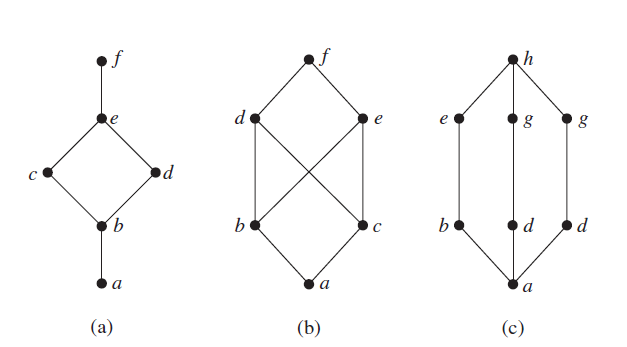# DM-Groups and Lattice-Q10Which of the above hasse diagrams (a),(b),(c) is/are is lattice?

(A).Only (a)

(B).Only (a) and (c)

(C).Only (b) and (c)

(D).All of (a),(b) and (c)asked Jul 8, 2019
reshown Jul 9, 2019

B is correct one
answered Jul 9, 2019 by (34,820 points)
for a hasse diagram to be lattice every pair of element must have unique LUB and GLB.

in diagram b

LUB(b,c)= d or e            GLB(d,e)=b,c

so diagram b is not lattice
answered Jul 9, 2019 by (9,260 points)
Yes This type of diagram called cross structure posetanswered Oct 5, 2019 by (200 points)